Vector Calculus - 8

# Vector Calculus - 8

Test Description

## 20 Questions MCQ Test Topic-wise Tests & Solved Examples for IIT JAM Mathematics | Vector Calculus - 8

Vector Calculus - 8 for Mathematics 2023 is part of Topic-wise Tests & Solved Examples for IIT JAM Mathematics preparation. The Vector Calculus - 8 questions and answers have been prepared according to the Mathematics exam syllabus.The Vector Calculus - 8 MCQs are made for Mathematics 2023 Exam. Find important definitions, questions, notes, meanings, examples, exercises, MCQs and online tests for Vector Calculus - 8 below.
Solutions of Vector Calculus - 8 questions in English are available as part of our Topic-wise Tests & Solved Examples for IIT JAM Mathematics for Mathematics & Vector Calculus - 8 solutions in Hindi for Topic-wise Tests & Solved Examples for IIT JAM Mathematics course. Download more important topics, notes, lectures and mock test series for Mathematics Exam by signing up for free. Attempt Vector Calculus - 8 | 20 questions in 60 minutes | Mock test for Mathematics preparation | Free important questions MCQ to study Topic-wise Tests & Solved Examples for IIT JAM Mathematics for Mathematics Exam | Download free PDF with solutions
 1 Crore+ students have signed up on EduRev. Have you?
Vector Calculus - 8 - Question 1

### If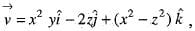then div curl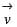is equal to

Vector Calculus - 8 - Question 2

### If Φ is a differentiable scalar function, then div grad Φ is equal to

Vector Calculus - 8 - Question 3

### If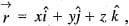then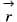is

Detailed Solution for Vector Calculus - 8 - Question 3

Explanation : curl F = ∇ × F

= (∂/∂x, ∂/∂y, ∂/∂z) × (F1, F2, F3)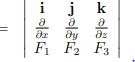(∂F3/∂y − ∂F2/∂z , ∂F1/∂z − ∂F3/∂x , ∂F2/∂x − ∂F1/∂y)

= (∂z/∂y − ∂y/∂z , ∂x/∂z − ∂z/∂x, ∂y/∂x − ∂x/∂y)

= (0, 0, 0)

Note that since this curl is 0, the radial vector field

F(x, y, z) = (x, y, z) is irrotational.

Vector Calculus - 8 - Question 4

The value of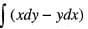along the circle x2 + y2 = 1 is

Vector Calculus - 8 - Question 5

The value of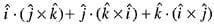is

Vector Calculus - 8 - Question 6

Directional derivative of ψ(x,y,z) = xy2 + 4xyz + z2 at the point (1, 2, 3) in the direction of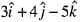is

Vector Calculus - 8 - Question 7

If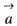and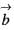are two vectors then the value of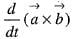will be

Vector Calculus - 8 - Question 8

The value of div grad Φ is

Vector Calculus - 8 - Question 9

The value of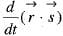is

Vector Calculus - 8 - Question 10

Gauss’s divergence theorem is

Vector Calculus - 8 - Question 11

If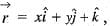then the value of div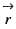will be

Vector Calculus - 8 - Question 12

Let W be the region bounded by the planes x = 0, y = 0, y = 3, z = 0 and x + 2z = 6. Let S be the boundary of this region. Using gauss’ divergence theorem, evaluate,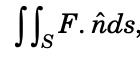where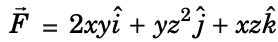and ň is the outward unit normal vector to S.

Detailed Solution for Vector Calculus - 8 - Question 12

By gauss divergence theorem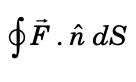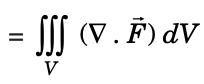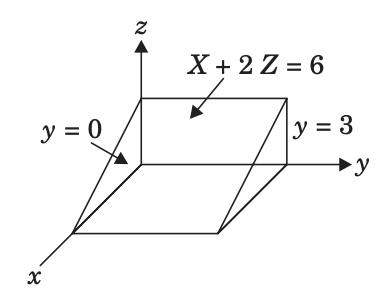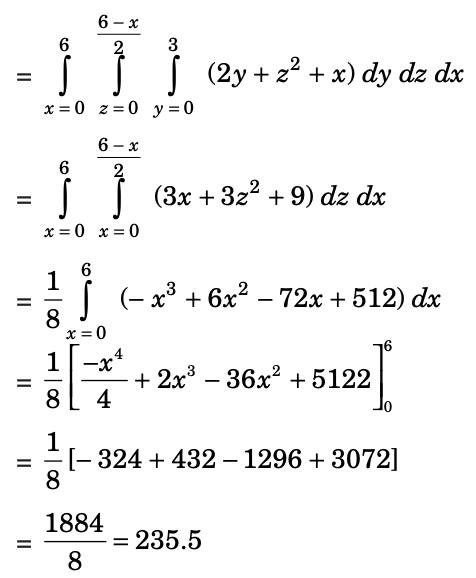Vector Calculus - 8 - Question 13

If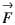is a differentiable vector point function, then the value of div curl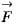is

Vector Calculus - 8 - Question 14

Let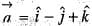and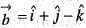Q. The unit vector perpendicular to the plane containing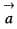and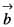is

Detailed Solution for Vector Calculus - 8 - Question 14

Given that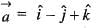and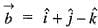Therefore, The unit vector perpendicular to the plane containing vectorandis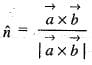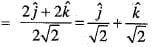Vector Calculus - 8 - Question 15

Let us consider the scalar point function f(x y, z) =x2 + y2 + z2
Q. The grad of f(x, y, z) is

Detailed Solution for Vector Calculus - 8 - Question 15

Grad f=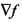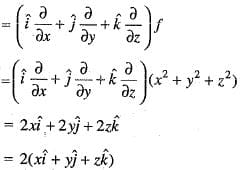Vector Calculus - 8 - Question 16

Let us consider the scalar point function f(x y, z) =x2 + y2 + z2
Q. The directional derivative of f(x, y, z) at the point P(1, 1, 1) along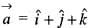is

Detailed Solution for Vector Calculus - 8 - Question 16

(Grad f)(1,1,1)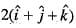Now, the directional derivative o f f at P(1,1,1) along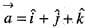is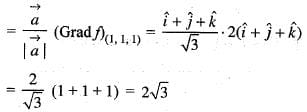Vector Calculus - 8 - Question 17

Let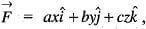where a, b and c are constants and S is the surface of unit sphere.
Q. The value of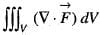is

Detailed Solution for Vector Calculus - 8 - Question 17

By Gauss divergence theorem,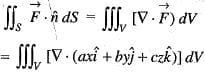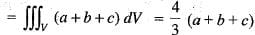Vector Calculus - 8 - Question 18

Letwhere a, b and c are constants and S is the surface of unit sphere.
Q. The value of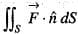is

Detailed Solution for Vector Calculus - 8 - Question 18

By Gauss divergence theorem,Since, V is enclosed by a sphere of unit radius. Thereofore
a + b + c = 1
and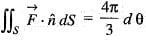Vector Calculus - 8 - Question 19

A vector normal to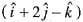is

Detailed Solution for Vector Calculus - 8 - Question 19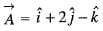We take,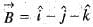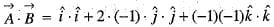= 1 - 2 + 1 = 0
So, B is normal to A.

Vector Calculus - 8 - Question 20

If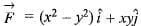and curve C is the arc of the curve y = x3 from (0,0) to (2,8), then the value of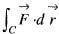Detailed Solution for Vector Calculus - 8 - Question 20

Since, C is the curve y = x3 from (0,0) to (2,8)
So, let x = t ⇒ y = t3
If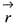is the position vector of any point on C, then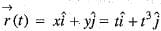or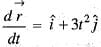or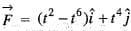At (0, 0) ⇒ t = x = 0 and at (2, 8) ⇒ t = 2
So,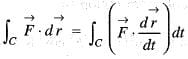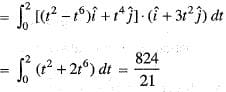## Topic-wise Tests & Solved Examples for IIT JAM Mathematics

27 docs|150 tests
 Use Code STAYHOME200 and get INR 200 additional OFF Use Coupon Code
Information about Vector Calculus - 8 Page
In this test you can find the Exam questions for Vector Calculus - 8 solved & explained in the simplest way possible. Besides giving Questions and answers for Vector Calculus - 8, EduRev gives you an ample number of Online tests for practice

## Topic-wise Tests & Solved Examples for IIT JAM Mathematics

27 docs|150 tests